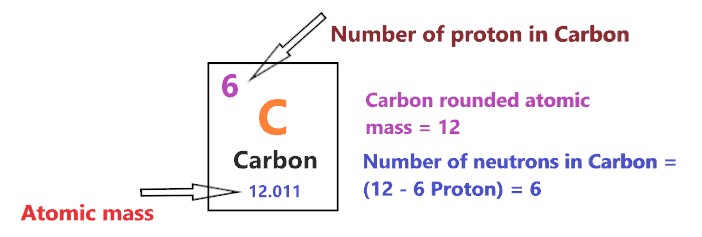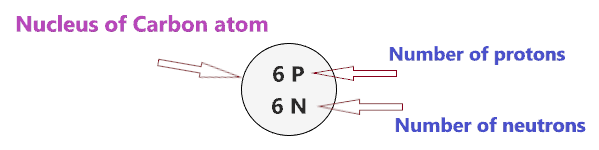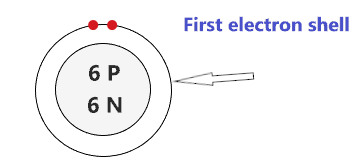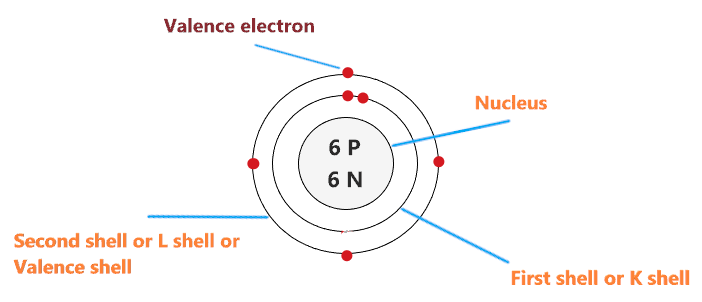# Bohr model of Carbon atom - How to draw Carbon(C) Bohr-Rutherford diagram?

Home  > Chemistry Article > Carbon Bohr model

The Bohr Model of Carbon(C) has a nucleus that contains 6 neutrons and 6 protons. This nucleus is surrounded by two-electron shells named K-shell and L-shell. The outermost shell in the Bohr diagram of Carbon contains 4 electrons that also called valence electrons.

 Name Carbon Bohr Model Number of neutrons 6 Number of protons 6 Number of electrons 6 Total electron shells 2 Electron in the First shell(K) 2 Electrons in the Second shell(L) 4 Total valence electrons in Carbon 4
Page Contents

## How to draw Bohr Model of Carbon(C)?

Bohr model describes the visual representation of orbiting electrons around the small nucleus. It used different electron shells such as K, L, M, N…so on. These shells hold a specific number of electrons, the electron shell which is closest to the nucleus has less energy and the electron shell which is farthest from the nucleus has more energy.

Bohr diagram is very interesting and easy to draw. Here, we will draw the Bohr diagram of the Carbon atom with some simple steps.

## Steps to draw the Bohr Model of Carbon atom

1. Find the number of protons, electrons, and neutrons in the Carbon atom

Protons are the positively charged particles and neutrons are the uncharged particles, both these are constituents of the atom nuclei. Electrons are the negatively charged particles that orbit the nucleus of an atom

To find the number of protons an atom contains, just look at their atomic number.

If the atomic number of atom is 7, then proton will also be 7, if atomic number is 8, then proton will also be 8.

So, the atomic number for Carbon is 6, hence, the number of protons in the Carbon atom is also 6.Now, to determine the number of neutrons in an atom, use this formula.

⇒ Number of neutrons in atom = Atomic mass of the atom(rounded to the nearest whole number) – Number of proton in an atom

For example, An atom have 10.811 atomic mass and 5 protons.

Then, to find the number of neutron, round the atomic mass to the near whole number, so, atomic mass 10.811 round to 11.

= (11 – 5 protons) = 6 number of neutrons in the atom

Now, To get the number of neutrons in a Carbon atom, look at its atomic mass which is 12.011 rounded to 12, and the number of protons in carbon is 6.

∴ Hence, the number of neutrons in Carbon atom = (12 – 6) = 6It should be noted that “The number of electrons in a neutral atom is equal to the number of protons”.

So, the Carbon atom is neutral, hence, its number of electrons will be equal to the number of protons which is Six as we already discussed.

⇒ The number of electrons in Carbon atom = 6

⇒ The number of protons in Carbon atom = 6

⇒ The number of neutrons in Carbon atom = 6

2. Draw the nucleus of an atom

A nucleus is a dense and small region that contains the number of protons and neutrons of an atom.

In this step, we have to draw a small circle that consists of a number of protons and the number of neutrons of a Carbon atom.3. Draw the First electron shell

“An electron shell may be thought of as an orbit followed by electrons around an atom’s nucleus.”

The first electron shell is also called the K-shell, this is the closest shell to the nucleus of an atom and can hold a maximum of two electrons.

As we know, the Carbon atom has a total of 6 electrons. So, put two electrons from it, in the first shell, next to each other.We have successfully drawn the first shell of the Carbon atom that can hold 2 electrons. As the Carbon atom has a total of 6 electrons, and from 6 electrons we have used two electrons in the first shell.

∴ (6 – 2) = 4 electrons

Therefore, we are left with 4 electrons, let’s put them in the next shells of the carbon atom.

4. Draw the Second electron shell

The second shell also called the L-shell that can hold a maximum of 8 electrons. This shell is drawn after the first electron shell.

Since we already used two electrons in the first shell, now, we have to put the remaining 4 electrons in the second shell.

In the second electron shell, the electrons are added one at a time, starting from the top position then going in a clockwise direction.

In second shell, electrons are added one at a time in clockwise direction as a clock position – 12 o’clock, 3 o’clock, 6 o’clock, 9 o’clock positions.So, we have to put 4 remaining electrons of the Carbon atom in the second shell, hence, start from the top position, put electrons one at a time, and go in a clockwise direction.### Bohr model of Carbon atom

That’s all, this is our Bohr model of the Carbon atom that contains 6 protons and 6 neutrons in the nucleus region, and 6 electrons are orbited around the nucleus, two electrons in the first shell, and four electrons in the second shell.

## Find Valence electron of Carbon through its Bohr diagram

Valence electrons are found in the outermost shell of an atom and they can take participate in the formation of a chemical bond. These electrons have more energy compare to the inner shell electrons.

From the Bohr diagram of an atom, we can easily find the number of valence electrons in an atom by looking at its outermost shell.

So, we have to find a valence electron in the Carbon atom, for this, look at its Bohr diagram.

Bohr’s diagram of Carbon has only two electron shells (K and L), the inner shell is K-shell and the outermost shell is L-shell.

Hence, the electrons found in the L-shell of the Carbon atom are its valence electrons because it is the outermost shell that also called the valence shell.

The L-shell or outer shell of the Carbon Bohr model contains 4 electrons, therefore, the number of valence electrons in the Carbon atom is 4.## Electron dot diagram of Carbon atom

Electron dot diagram also called lewis structure which represents the valence electrons of atoms.

As, from the Bohr diagram of Carbon, we got to know, it has 4 valence electrons.

So, just represent these 4 valence electrons around the Carbon atom as a dot.## The electron configuration of Carbon

Electron configuration is the distribution of electrons of an atom or molecule in atomic or molecule orbitals”

Carbon has an atomic number of 6 and it contains a total number of 6 electrons. From the Bohr model of Carbon, we know, 2 electrons are in the K-shell and 4 electrons are in the L-shell.

So, based on the shell, the electronic configuration of the Carbon atom is [2, 4].

Or the electronic configuration of Carbon is 1s22s22p2 since it contains a total of 6 electrons, the first two-electron will go in 1s orbital, the next two in 2s orbital, and the remaining two electrons will go in 2p orbital.

S orbital can hold maximum of 2 electrons and P orbital can hold maximum of 6 electrons.## FAQ

### How many electron shells a Carbon Bohr model contains?

Electron shell also called energy level, you can find the number of electron shells for an element by knowing its period number in the periodic table.

The elements or atoms in the first period of the periodic table have one energy level or one electron shell, same as, the elements in the second period have two energy levels or two-electron shells and so on…

So, the Carbon atom belongs to the 2nd Period in the periodic table, hence, the number of electron shells for the Bohr model of carbon will be 2 (K-shell and L-shell).

### What is the outer shell of the Bohr diagram of the Carbon atom?

The outermost shell also called the valence shell, which contains valence electrons of the atom.

According to the Bohr diagram of Carbon, the outer shell is L-shell which contains 4 valence electrons.

## Properties of Carbon

1. It belongs to Group 14 and period 2 in the periodic table.
2. It is nonmetallic and tetravalent in nature.
3. It has an electronegativity of 2.55 according to the Pauling scale.
4. The common oxidation state of carbon is +4 and +2.
5. Carbon is sometimes referred to as the “Kings of the elements”.
6. The two common allotropes of carbon are Graphite and Diamond.

## Summary

• The Bohr model of Carbon is drawn with only two electron shells, the first shell contains 2 electrons and the second shell contains 4 electrons.
• Carbon is neutral and its atomic number is 6, hence, the number of protons and electrons available for its Bohr diagram is also 6.
• The number of neutrons for the Bohr diagram of Carbon can be found by subtracting the number of protons from the atomic mass(rounded to the nearest whole).
• The electron configuration of carbon in terms of the shell is [2, 4], or in normal form, it is [He] 2s2 2p2.
##### Subscribe to Blog via Email

Join 2 other subscribers

Share it...